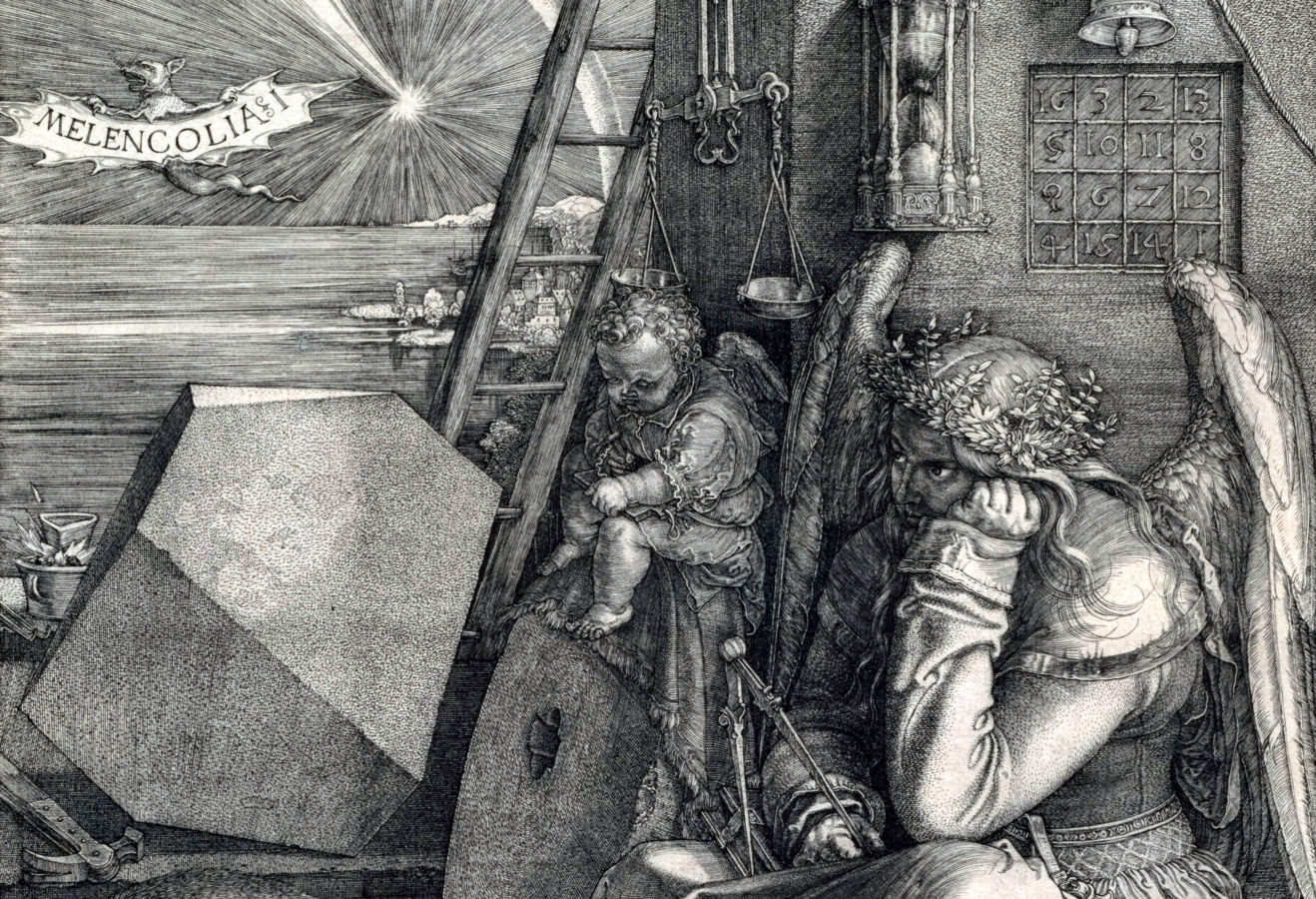Connect with us

#### The Mystery Of Dürer’s Magic SquareAlbrecht Dürer, Melencolia I, 1514, National Gallery of Art, Washington, DC, USA. Detail.

# The Mystery Of Dürer’s Magic Square

Many weird things appear in Albrecht Dürer’s famous engraving Melencolia. Among them is a magic constant 34 square, a well-known and enigmatic example. The engraving shows a disorganized jumble of scientific equipment lying unused while an intellectual sits absorbed in thought. ‘Dürer’s magic square’ is located in the upper right-hand corner of the engraving. The numbers 15 and 14 appear in the middle of the bottom row, indicating the date of the engraving, 1514.

The sum 34 can be found in the rows, columns, diagonals, each of the quadrants, the center four squares, the corner squares, the four outer numbers clockwise from the corners (3+8+14+9), and likewise, the four counter-clockwise, the two sets of four symmetrical numbers (2+8+9+15 and 3+5+12+14), the sum of the middle two entries of the two outer columns and rows (e.g. 5+9+8+12), as well as several kite-shaped quartets, e.g. 3+5+11+15. Actually, there are 86 different combinations of four numbers from Dürer’s square that add up to the sum of the magic number, 34!

Each row, each column, and each diagonal adds up to 34; these are the traditional magic properties. But there is more magic to be found here. There are actually thirteen different ways of dividing this square into four groups of four cells, with each group of four cells adding to 34. The menu in the applet can be used to select among these. The positions in the ‘Dürer square’ can be seen as a finite vector space in which each set of four groups of four cells is a set of parallel affine planes.

‘Dürer’s magic square’ has additional properties: the sum in any of the four quadrants, as well as the sum of the middle four numbers, are all 34. It is thus a gnomon magic square. A gnomon magic square is a 4×4 magic square in which the elements in each 2×2 corner have the same sum. In addition, any pair of numbers symmetrically placed about the center of the square sum to 17, making the square even more magical.